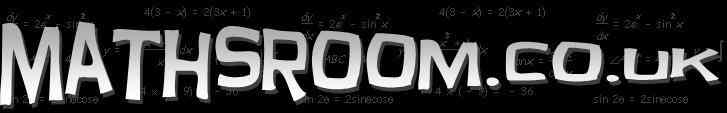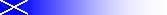Online interactive resources for the Scottish Maths curriculum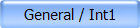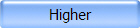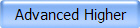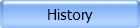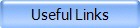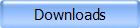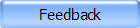Credit / Int2 Exam Tips Helpful tips and things to avoid in your Maths exam.  Standard Grade Maths Exam Solutions Credit 2002 - 2006 BBC Bitesize – Standard Grade Maths I Number, Pythagoras, Trigonometry, Algebra, Areas & Volumes, Graphs BBC Bitesize – Standard Grade Maths II Trigonometry, Algebra, Statistics Credit Course Revision A variety of links from the wonderful Madras College website. GCSE Revision Many topics suitable for revision at Credit level.Understanding Payslips Detailed explanation of each section on an average payslip. Distance-Time 1 (Java Applet) Create real-time D-T graph by controlling the movement of a wee man. Distance-Time 2 (Java Applet) Control the speed, direction and starting position of two runners. Watch them race then analyse the Distance-Time graph. Also includes questions and points for discussion. Distance-Time 3 (Flash) Excellent Distance, Speed & Time activity in the context of a football match. Includes video footage of Man Utd vs West Ham (Nov 2000) Scientific Notation (Flash) Explore the components of scientific notation. Includes exercises on converting between forms. Circle Geometry 1 (Java Applet) An angle inscribed in a semi-circle is a right angle. Circle Geometry 2 (Java Applet) Explore tangents to circles.Solving Equations Practice Solve randomly generated equations, for example: 2x = -6, 5(x + 2) = 15, 4x - 1 = -x + 9. Working should be done on paper and answer inputed for correction. Gradient Calculation (Shockwave) Determine the gradient from two user-defined points. y = mx + c 1 (Java Applet) Watch the line change in real time as you alter m or c. y = mx + c 2 (Shockwave) Experiment with the m and c and see how the gradient of a line is calculated. y = mx + c 3 (Java Applet) Anothere page experimenting with the m and c to see how the gradient of a line is calculated. Simultaneous Equations (Java Applet) Graphs two linear equations showing the solution as the point where the lines intersect. Quadratic Equation Proof (Flash) Arrange the lines of the proof into the correct order. Zap It! Game (Shockwave) Try to adjust the quadratic function, y=ax²+bx+c, to 'Zap' as many data points as possible.Trigonometry 1 (Flash) Animated introduction to right-angled trigonometry. Trigonometry 2 (Java Applet) Explore the signs and magnitudes of the trig ratios. Radian measure used. Trigonometry 3 (Flash) ASTC demonstration Trigonometry 4 (Shockwave) The Unit Circle. Explore the relationship between the unit circle and the graphs of the sine, cosine, and tangent functions. Trigonometry 5 On-line Test. Includes Arc Length, Sector Area, Sine & Cosine Rules. Trigonometry 6 (Shockwave) Shifting and Scaling Sine and Cosine Curves. Experiment with the graphs of trigonometric functions of the form y = a sin[b (x - c)] + d. Relate the equation and graph to amplitude, period, and frequency. Histogram  Bar Graph  Pie Chart  Stem & Leaf Diagram  Box Plot (Java Applet) Interactive Statistical Diagrams View and create these using built in data or user defined data.